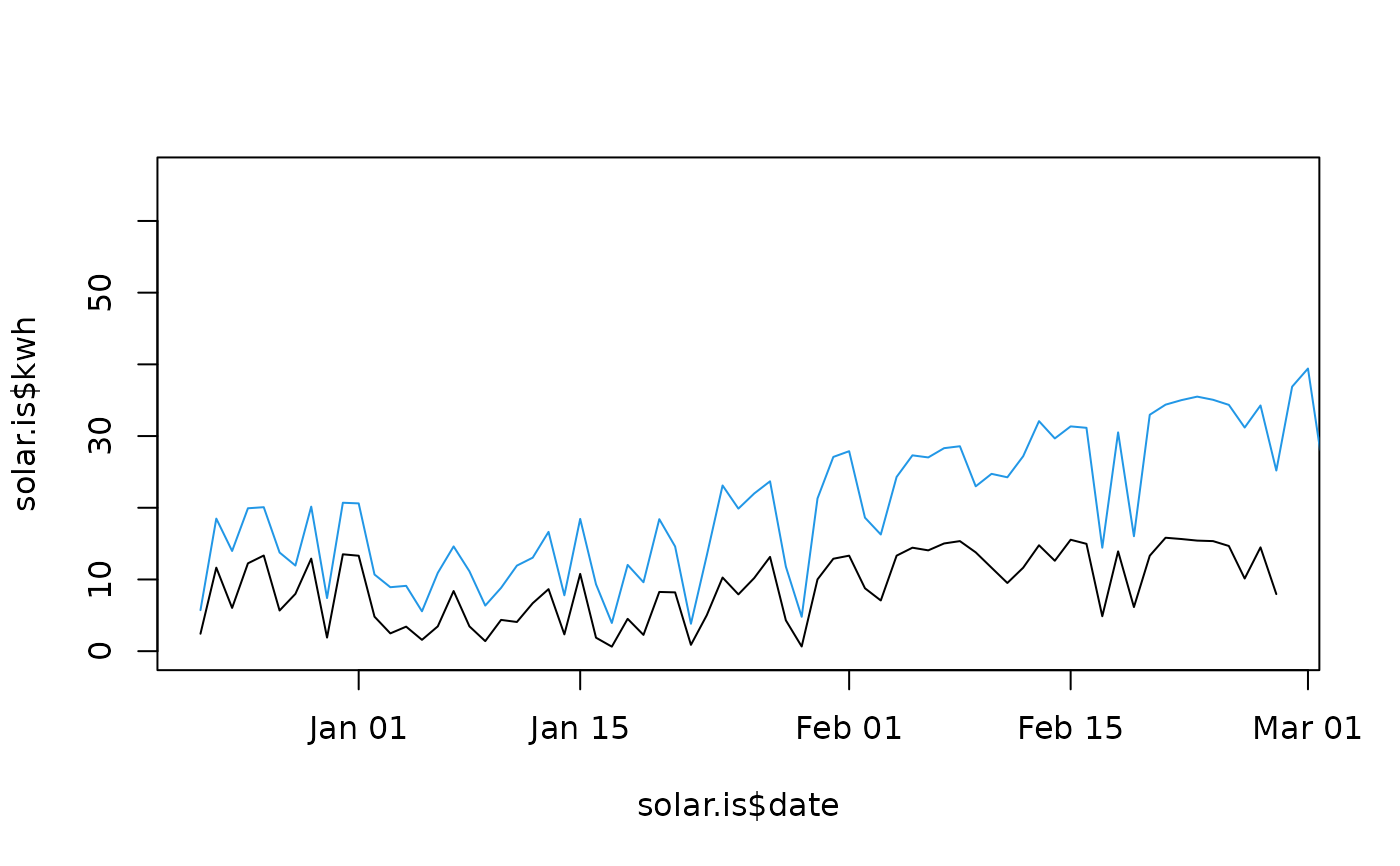The data provide the energy output for several months from two roof-top solar arrays in San Francisco. This city is known for having highly variable weather, so while these two arrays are only about 1 mile apart from each other, the Inner Sunset location tends to have more fog.

## Usage

solar

## Format

A data frame with 284 observations on the following 3 variables. Each row represents a single day for one of the arrays.

location

Location for the array.

date

Date.

kwh

Number of kWh

## Source

These data were provided by Larry Rosenfeld, a resident in San Francisco.

The Haight-Ashbury array is a 10.4 kWh array, while the Inner Sunset array is a 2.8 kWh array. The kWh units represents kilowatt-hours, which is the unit of energy that typically is used for electricity bills. The cost per kWh in San Francisco was about $0.25 in 2016. ## Examples  solar.is <- subset(solar, location == "Inner_Sunset") solar.ha <- subset(solar, location == "Haight_Ashbury") plot(solar.is$date, solar.is$kwh, type = "l", ylim = c(0, max(solar$kwh)))
lines(solar.ha$date, solar.ha$kwh, col = 4)d <- merge(solar.ha, solar.is, by = "date")
plot(d$date, d$kwh.x / d\$kwh.y, type = "l")